# Quantitative Aptitude Quiz For IBPS RRB PO, Clerk Prelims 2021- 16th April

Direction (1-5): In the following questions, two equations numbered I and II are given. You have to solve both the equations and give answers among the following options.
(a) if x>y
(b) if x≥y
(c) if x<y
(d) if x ≤y
(e) if x=y or the relationship cannot be established.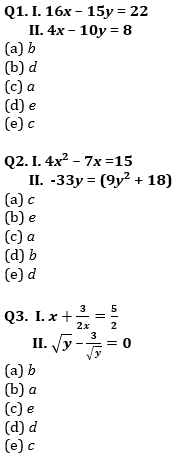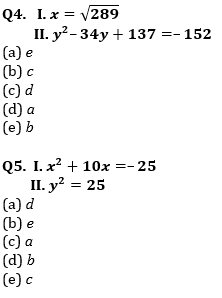(a) x > y
(b) x ≥ y
(c) x < y
(d) x ≤ y
(e) x = y or no relation can be established between x and y.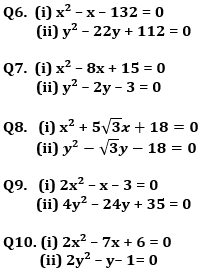Directions (11-15): In each question two equations numbered I and II are given. You have to solve both the equations and mark appropriate answer.
(a) If x<y
(b) If x>y
(c) If x≥y
(d) If x≤y
(e) If x=y or no relation can be established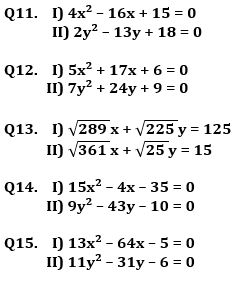Practice More Questions of Quantitative Aptitude for Competitive Exams:

###### Study Plan for IBPS RRB PO/Clerk Prelims 2021

Solutions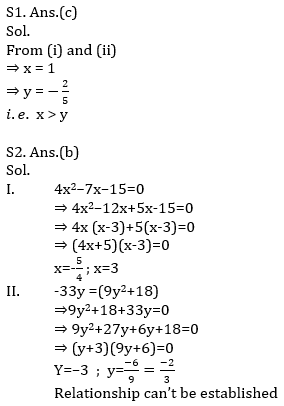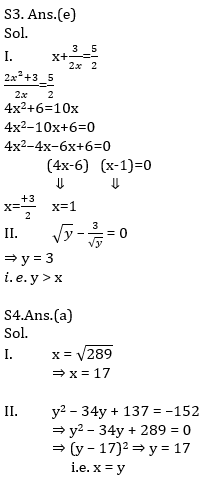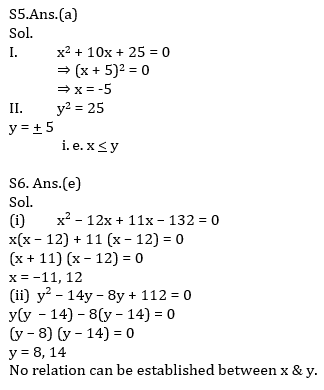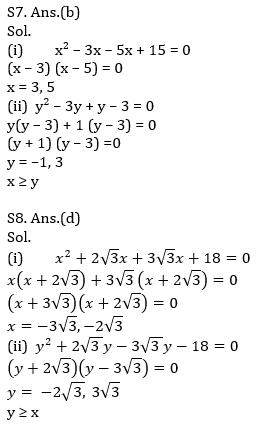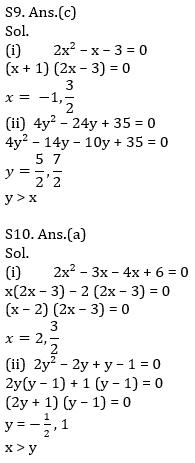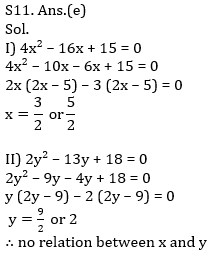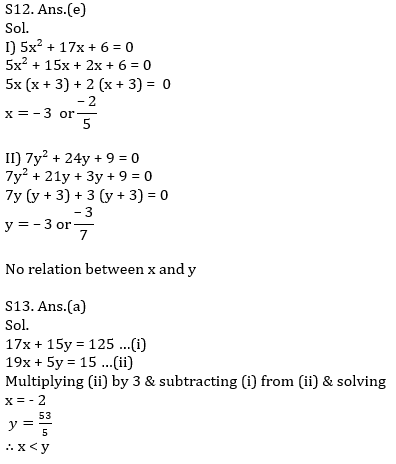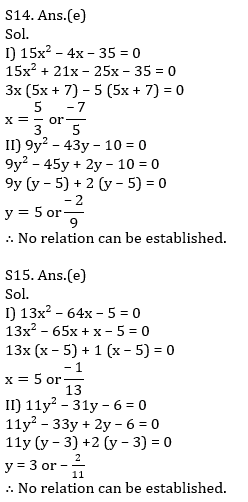×

Thank You, Your details have been submitted we will get back to you.
×
OR

×
OR